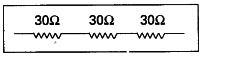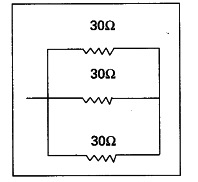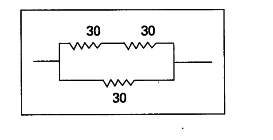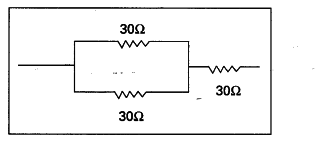# Imagine that you have three resistors each of value 30 Ω

Imagine that you have three resistors each of value 30 Ω. How many resistors can you obtained by connecting these three in different ways ? Draw the relevant diagrams.

I can obtain the following resistors by various combinations.
i) When the three resistors are connected in series
{{R}_{eq}} = R1 + R2 + R3
= 30+ 30+ 30 = 90 Ω.ii) If all the three resistors are connected in parallel
1/ {{R}_{eq}} = 1/r1 + 1/r2 + 1/r3
= 1/30 + 1/30+ 1/30
=1+1+1/30 = 3/30 = 1/10
{{R}_{eq}} = 10 Ω.iii) If two resistors are connected in series and the third resistor is connected in parallel to them.
{{R}_{eq}} for series = R1 + R2
= 30+ 30 = 60 Ω.
{{R}_{eq}} for 60 Ω, 30 Ω in parallel
1/{{R}_{eq}} = 1/60 + 1/30 = 1+2/30 = 3/60 = 1/20
{{R}_{eq}} = 20 Ωiv) If two resistors are connected in parallel and third resistor is connected in series.
1/{{R}_{eq}} for parallel connection =1/r1 + 1/r2
= 1/30 + 1/30 = 1+1/30 = 2/30 =1/15
{{R}_{eq}} = 15 Ω
{{R}_{eq}} for series combination of 15 Ω and 30 Ω
{{R}_{eq}} = R1 + R2 = 15+30 = 45 Ω